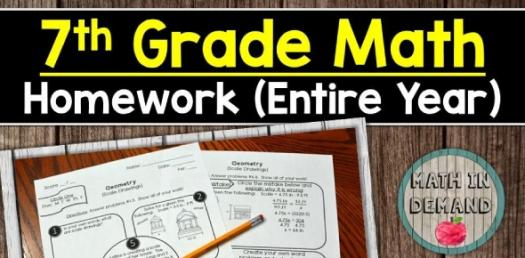# Math Formula's - 7th Grade Math

Approved & Edited by ProProfs Editorial Team
The editorial team at ProProfs Quizzes consists of a select group of subject experts, trivia writers, and quiz masters who have authored over 10,000 quizzes taken by more than 100 million users. This team includes our in-house seasoned quiz moderators and subject matter experts. Our editorial experts, spread across the world, are rigorously trained using our comprehensive guidelines to ensure that you receive the highest quality quizzes.
| By Samprevost
S
Samprevost
Community Contributor
Quizzes Created: 1 | Total Attempts: 454
Questions: 9 | Attempts: 454SettingsRead and find out :D! * ^ is a exponent. Wen u type an answer and pi is involved use (pi)!

• 1.

### Perimeter Of A Square

• A.

S^2

• B.

4s

B. 4s
Explanation
The formula for finding the perimeter of a square is 4s, where s represents the length of one side of the square. Since a square has four equal sides, multiplying the length of one side by 4 gives us the total length of all four sides combined. Therefore, the correct answer is 4s.

Rate this question:

• 2.

### Circumference

2(pi)r
(pi)d
Explanation
The formula for finding the circumference of a circle is given by 2(pi)r or (pi)d. Here, "r" represents the radius of the circle and "d" represents the diameter. Both formulas are correct and can be used interchangeably to calculate the circumference. The first formula, 2(pi)r, calculates the circumference by multiplying the radius by 2 and then multiplying it by pi. The second formula, (pi)d, calculates the circumference by directly multiplying the diameter by pi. Therefore, both formulas yield the same result and can be used depending on the given information.

Rate this question:

• 3.

• 4.

### Area of a rectangle

a= lw
lw
A=lw
Explanation
The given expressions "a= lw", "lw", and "A=lw" represent the formula for finding the area of a rectangle. In this formula, "l" represents the length of the rectangle and "w" represents the width. The expression "a= lw" indicates that the area of the rectangle is equal to the product of its length and width. The expression "lw" is another way of representing the same formula. Lastly, "A=lw" is a shorthand notation where "A" represents the area and is equal to the product of the length and width of the rectangle.

Rate this question:

• 5.

### Area of a parallelogram

• A.

Bh

• B.

Lwh

• C.

???

• D.

Does that exist?

A. Bh
Explanation
The formula for finding the area of a parallelogram is given by multiplying the base (b) by the height (h). Therefore, the correct answer is bh. The other options mentioned, lwh and "does that exist?", are incorrect and do not represent the correct formula for finding the area of a parallelogram.

Rate this question:

• 6.

### Area of a triangle

• A.

Bh

• B.

Lwh

• C.

Bh

• D.

(pi)r^2

• E.

1/2bh

E. 1/2bh
Explanation
The formula for finding the area of a triangle is 1/2bh, where b is the base of the triangle and h is the height. This formula is derived from the fact that the area of a triangle is equal to half the product of its base and height. Therefore, the correct answer is 1/2bh.

Rate this question:

• 7.

### Area of a trapezoid

• A.

1/2h(b1+b2)

• B.

Bh

• C.

Lwh

• D.

Uhmmm

• E.

(pi)r^2

A. 1/2h(b1+b2)
Explanation
The formula for finding the area of a trapezoid is 1/2 multiplied by the height of the trapezoid, multiplied by the sum of the lengths of its parallel sides (b1 and b2). This formula is derived from splitting the trapezoid into a rectangle and two right triangles, and then finding the area of each shape and summing them.

Rate this question:

• 8.

### Area of a circle

(pi)r^2
Explanation
The formula to calculate the area of a circle is given by (pi)r^2, where r represents the radius of the circle. This formula is derived from the fact that the area of a circle is equal to the product of pi (approximately 3.14159) and the square of its radius. Therefore, the correct answer is (pi)r^2.

Rate this question:

• 9.

### Volume of a prismvolume of a cylinderPythagorean theorem simple interest

Related TopicsBack to top